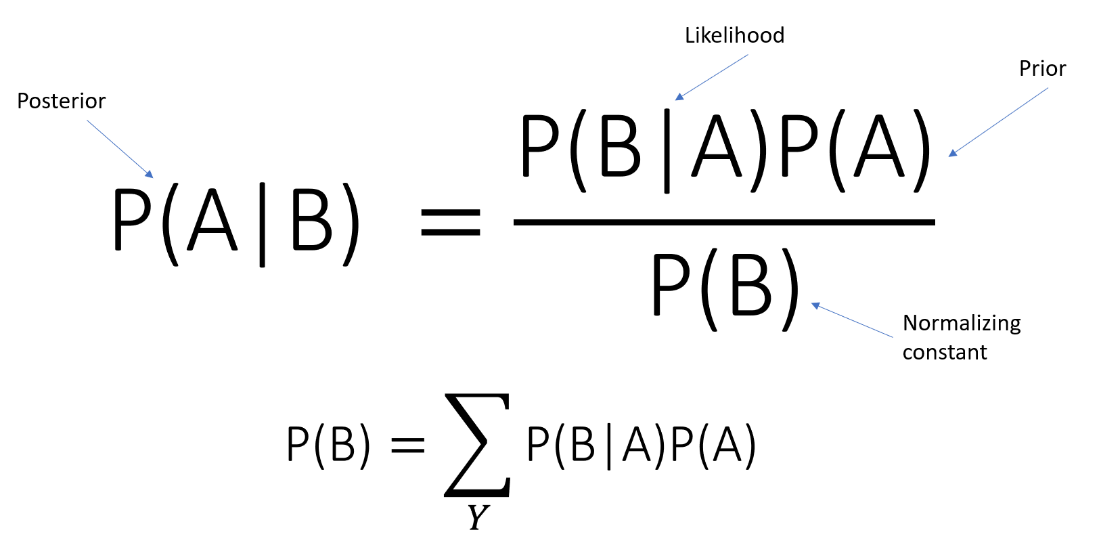/  Top Machine learning interview questions and answers   /  What is the difference between Prior Probability and Posterior Probability?## What is the difference between Prior Probability and Posterior Probability?

### Prior Probability

A prior probability is the probability that an observation will fall into a group before you collect the data. The prior is a probability distribution that represents your uncertainty over θ before you have sampled any data and attempted to estimate it – usually denoted π(θ).

### Posterior Probability

A posterior probability is the probability of assigning observations to groups given the data. The posterior is a probability distribution representing your uncertainty over θ after you have sampled data – denoted π(θ|X). It is a conditional distribution because it conditions on the observed data.

From Bayes’ theorem we relate the two: